# The Region Class

A region describes the interior of a closed graphics shape, or form. A form has two areas: a nonclient area and a client area. The nonclient area (which does not allow for user-drawn graphics objects) includes the title barand, depending on the application, horizontal and vertical scroll bars. This area cannot be used to draw graphics objects. The client area is used to draw controls and graphics objects.

In the .NET Framework library, the Region class object represents a region. If you have ever developed a complex .NET graphics application that requires a lot of rendering, you may have used this object a lot.

6.2.1 Constructing a Region Object

The Region class provides five overloaded forms. Using these forms, you can construct a Region object from a Rectangle, RectangleF, GraphicsPath, or RegionData object, or with no parameters. The following code snippet creates Region objects in different ways using different arguments.

```
```
```// Create two rectangles
Rectangle rect1 =
new Rectangle(20, 20, 60, 80);
RectangleF rect2 =
new RectangleF(100, 20, 60, 100);
// Create a graphics path
GraphicsPath path = new GraphicsPath();
// Add a rectangle to the graphics path
// Create a region from rect1
Region rectRgn1 = new Region(rect1);
// Create a region from rect2
Region rectRgn2 = new Region(rect2);
// Create a region from GraphicsPath
Region pathRgn = new Region(path);
```
```
```

The Region class has no properties. After constructing a region, an application can use the Graphics class's FillRegion method to fill the region.

Table 6.1 describes the methods of the Region class briefly. They are discussed in detail in Section 6.2.2 through 6.2.4

6.2.2 The Complement, Exclude, and Union Methods

We saw the Region class methods in Table 6.1. Now let's use these methods in our applications.

The Complement method updates the portion of a Region object (specified by a rectangle or a region) that does not intersect the specified region. It takes an argument of type Rectangle, RectangleF, GraphicsPath, or Region and updates the region. Listing 6.7 creates two Region objects and draws rectangles with different pens. The Complement method updates only the portion of the first region that falls within the second region.

Listing 6.7 Using the Complement method of the Region class

```// Create Graphics object
Graphics g = this.CreateGraphics();
// Create two rectangles
Rectangle rect1 = new Rectangle(20, 20, 60, 80);
Rectangle rect2 = new Rectangle(50, 30, 60, 80);
// Create two regions
Region rgn1 = new Region(rect1);
Region rgn2 = new Region(rect2);
// Draw rectangles
g.DrawRectangle(Pens.Green, rect1);
g.DrawRectangle(Pens.Black, rect2);
// Complement can take Rectangle, RectangleF,
// Region, or GraphicsPath as an argument
rgn1.Complement(rgn2);
// rgn1.Complement(rect2);
g.FillRegion(Brushes.Blue, rgn1);
// Dispose of object
g.Dispose();
```

Figure 6.5 shows the output from Listing 6.7. Our code updates a portion of rgn1 that doesn't intersect with rgn2. It is useful when you need to update only a specific part of a region. For example, suppose you're writing a shooting game application and your program updates the targets only after gunfire. In this scenario you need to update only the target region, not the entire form.

Figure 6.5. Complementing regions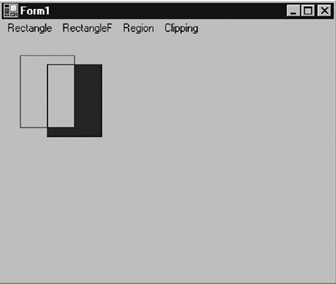Table 6.1. Region methods

Method

Description

Clone

Creates an exact copy of a region.

Complement

Updates a region to the portion of a rectangle that does not intersect with the region.

Exclude

Updates a region to the portion of its interior that does not intersect with a rectangle.

FromHrgn

Creates a new Region object from a handle to the specified existing GDI region.

GetBounds

Returns a RectangleF structure that represents a rectangle that bounds a region.

GetHrgn

Returns a window handle for a region.

GetRegionData

Returns a RegionData object for a region. RegionData contains information describing a region.

GetRegionScans

Returns an array of RectangleF structures that approximate a region.

Intersect

Updates a region to the intersection of itself with another region.

IsEmpty

Returns true if a region is empty; otherwise returns false.

IsInfinite

Returns true if a region has an infinite interior; otherwise returns false.

IsVisible

Returns true if the specified rectangle is contained within a region.

MakeEmpty

Marks a region as empty.

MakeInfinite

Marks a region as infinite.

Transform

Applies the transformation matrix to the region.

Translate

Offsets the coordinates of a region by the specified amount.

Union

Updates a region to the union of itself and the given graphics path.

Xor

Updates a region to the union minus the intersection of itself with the given graphics path.

The Exclude method updates the part of a region that does not interact with the specified region or rectangle. Like Complement, Exclude takes an argument of type Rectangle, RectangleF, GraphicsPath, or Region and updates the region. Listing 6.8 creates two Region objects and draws rectangles with different pens, then calls Exclude.

Listing 6.8 Using the Exclude method of the Region class

```Rectangle rect1 = new Rectangle(20, 20, 60, 80);
Rectangle rect2 = new Rectangle(50, 30, 60, 80);
Region rgn1 = new Region(rect1);
Region rgn2 = new Region(rect2);
g.DrawRectangle(Pens.Green, rect1);
g.DrawRectangle(Pens.Black, rect2);
rgn1.Exclude(rgn2);
g.FillRegion(Brushes.Blue, rgn1);
```

Figure 6.6 shows the output from Listing 6.8. Only the excluded part of the region is updated.

Figure 6.6. Excluding regions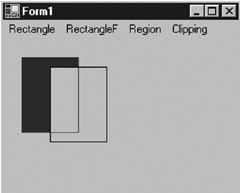From the code of Listing 6.8, replacing the line

```
```
```rgn1.Exclude(rgn2);
```
```
```

with

```
```
```rgn1.Union(rgn2);
```
```
```

produces Figure 6.7, which updates the union of both regions (or rectangles). Like Exclude and Complement, the Union method can take Rectangle, RectangleF, GraphicsPath, or Region as an argument.

Figure 6.7. Applying Union on regions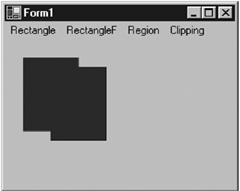6.2.3 The Xor and Intersect Methods

The Xor method updates the union of both regions (or rectangles) except the intersection area of the rectangle itself. Replacing Exclude with Xor, as shown in Listing 6.9, generates Figure 6.8.

Figure 6.8. Using the Xor method of the Region class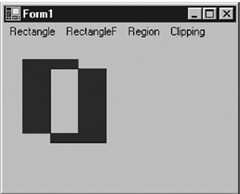Listing 6.9 Using the Xor method of the Region class

```// Create Graphics object
Graphics g = this.CreateGraphics();
g.Clear(this.BackColor);
// Create rectangles
Rectangle rect1 = new Rectangle(20, 20, 60, 80);
Rectangle rect2 = new Rectangle(50, 30, 60, 80);
// Create regions
Region rgn1 = new Region(rect1);
Region rgn2 = new Region(rect2);
// Draw rectangles
g.DrawRectangle(Pens.Green, rect1);
g.DrawRectangle(Pens.Black, rect2);
// Xor two regions
rgn1.Xor(rgn2);
// Fill the region after Xoring
g.FillRegion(Brushes.Blue, rgn1);
// Dispose of object
g.Dispose();
```

The Intersect method is the reverse of Xor. It updates only the intersection region of two regions or rectangles. For example, if you replace line

```
```
```rgn1.Xor(rgn2);
```
```
```

with the following code:

```
```
```rgn1.Intersect(rgn2);
```
```
```

the new output will look like Figure 6.9.

Figure 6.9. Using the Intersect method of the Region class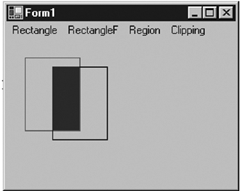6.2.4 GetBounds and Other Methods

The IsEmpty method takes a Graphics object as an argument and returns true if a region is empty. Otherwise it returns false. IsInfinite returns true if a region is infinite (otherwise it returns false), and it takes a Graphics object as the only argument.

The MakeEmpty and MakeInfinite methods make a region empty or infinite, respectively. An infinite region completely covers the area of a control.

The GetBounds method returns the bounds of a region. This method also takes a Graphics object as an argument.

The code in Listing 6.10 uses these methods. It makes rgn2 infinite and fills it with a red pen, which fills the entire form with red.

Listing 6.10 Using GetBounds and other methods of the Region class

```// Create a Graphics object
Graphics g = this.CreateGraphics();
g.Clear(this.BackColor);
// Create rectangles and regions
Rectangle rect1 =
new Rectangle(20, 20, 60, 80);
Rectangle rect2 =
new Rectangle(50, 30, 60, 80);
Region rgn1 = new Region(rect1);
Region rgn2 = new Region(rect2);
// If region is not empty, empty it
if (! rgn1.IsEmpty(g))
rgn1.MakeEmpty();
// If region is not infinite, make it infinite

if (! rgn2.IsInfinite(g))
rgn2.MakeInfinite();
// Get bounds of the infinite region
RectangleF rect = rgn2.GetBounds(g);
// Display
MessageBox.Show(rect.ToString());
// Fill the region
g.FillRegion(Brushes.Red, rgn2);
// Dispose of object
g.Dispose();
```

An infinite region's starting coordinates are negative numbers, and its height and width are large positive numbers, as Figure 6.10 shows. Using FillRegion on an infinite region fills the entire form.

Figure 6.10. Bounds of an infinite regionGDI+ Programming with C#
ISBN: 073561265X
EAN: N/A
Year: 2003
Pages: 145